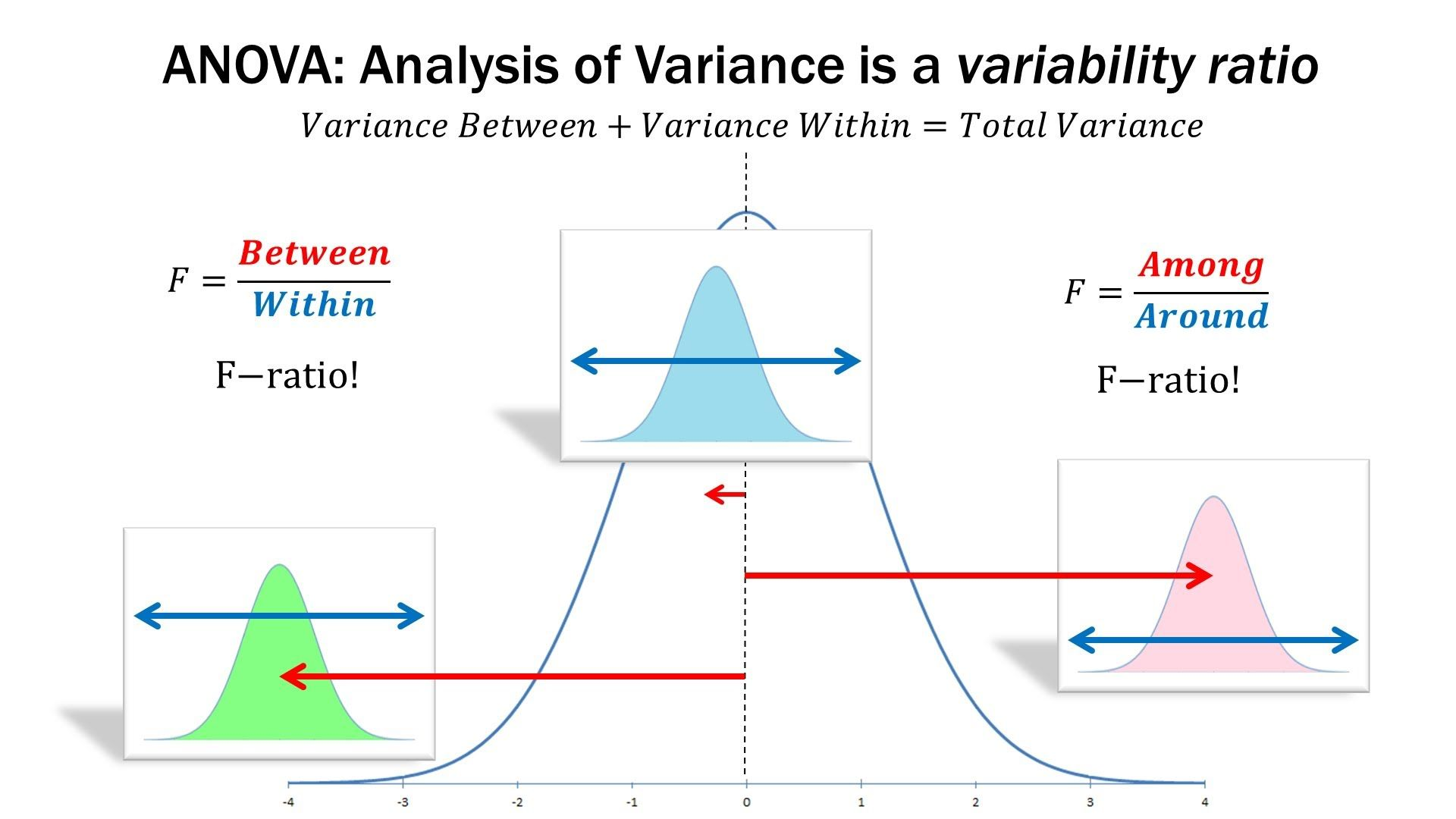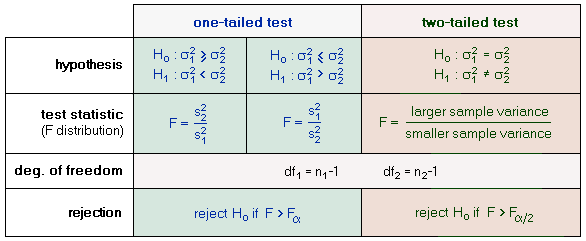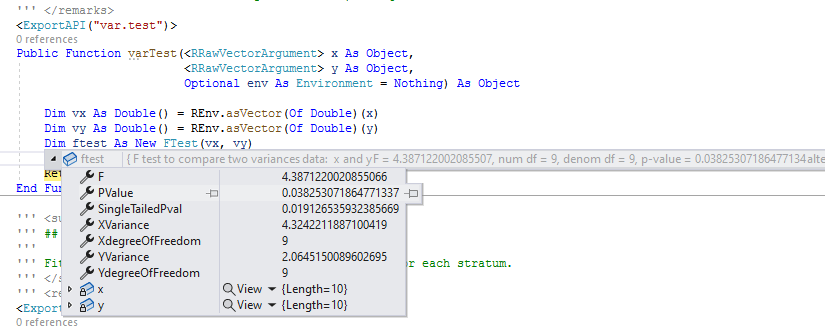## F检验

F检验也可以称为方差齐性检验。它是一种在零假设（null hypothesis, H0）之下，统计值服从F-分布的检验。其通常是用来分析用了超过一个参数的统计模型，以判断该模型中的全部或一部分参数是否适合用来估计母体。F检验的实现非常的简单：我们将两个待检验的向量的方差相除即可得到F统计值。然后再通过得到的F统计值结果，基于两个向量的自由度进行查表操作既可以得到对应的F检验的Pvalue了。Public ReadOnly Property F As Double
Get
Dim q As Double = XVariance ^ 2
Dim p As Double = YVariance ^ 2
Return q / p
End Get
End Property

Public ReadOnly Property SingleTailedPval As Double
Get
Dim f As Double = Me.F
Dim single_tailed_pval As Double = Distribution.FDistribution(
fValue:=f,
freedom1:=XdegreeOfFreedom,
freedom2:=YdegreeOfFreedom
)
Return single_tailed_pval
End Get
End Property

Public ReadOnly Property PValue As Double
Get
Return SingleTailedPval * 2
End Get
End Property## ANOVA检验H0: μ1 = μ2 = μ3 ... = μk
H1: Means are not all equal.

observations = data.frame(
v     = [
2, 3, 7, 2, 6,
10, 8, 7, 5, 10, 10, 8, 7, 5, 10,
2, 3, 7, 2, 6
],
group = append(rep("A", 5), append(rep("B", 10), rep("C", 5)))
);

### 组内平均值计算

Dim total As Double = 0
Dim observationsCount = 0

For i As Integer = 0 To groups.Count - 1
Dim g = groups(i)
Dim groupTotal As Double = 0

For j As Integer = 0 To g.ary.Length - 1
groupTotal += g.ary(j)
Next

total += groupTotal
observationsCount += g.ary.Length
g.mean = groupTotal / g.ary.Length
Next

allObservationsMean = total / observationsCount

### 组内方差计算

For i As Integer = 0 To groups.Count - 1
Dim g As Group = groups(i)

For j As Integer = 0 To g.ary.Length - 1
Dim observation = g.ary(j)
Dim result = observation - g.mean

Next

SSW_sum_of_squares_within_groups += g.raisedSum
Next

### 总方差计算

SS_total_sum_of_squares = 0

For i As Integer = 0 To groups.Count - 1
Dim g As Group = groups(i)

For j As Integer = 0 To g.ary.Length - 1
Dim observation = g.ary(j)
Dim result = observation - allObservationsMean

SS_total_sum_of_squares += stdNum.Pow(result, 2)
Next
Next

SSB_sum_of_squares_between_groups = SS_total_sum_of_squares - SSW_sum_of_squares_within_groups

### 平均方差计算

Dim observations = 0

numenator_degrees_of_freedom = groups.Count - 1
SSB = SSB_sum_of_squares_between_groups / numenator_degrees_of_freedom

For Each g In groups
observations += g.ary.Length
Next

' degrees_of_freedom = observations - groups.size();
denomenator_degrees_of_freedom = observations - groups.Count
SSW = SSW_sum_of_squares_within_groups / denomenator_degrees_of_freedom• SSB: 这是每组平均值与总平均值之间的平方差的总和乘以每组元素的数量。
• SSW: 这是组均值与组中每个值之间的平方差的总和。

### F检验以及P-value计算

' anova.fScore_determineIt()
Public Overridable Function fScore_determineIt() As Double
F_score = SSB / SSW
singlePvalue = New FDistribution(numenator_degrees_of_freedom, denomenator_degrees_of_freedom).GetCDF(F_score)
doublePvalue = singlePvalue * 2

Return F_score
End Function## 代码模块测试observations = data.frame(
v     = [
20, 3, 7, 2, 6,
10, 8, 7, 5, 10, 10, 8, 7, 5, 10,
2,  3, 7, 2, 6
],
group = append(rep("A", 5), append(rep("B", 10), rep("C", 5)))
);

print(observations, max.print = 6);
#                v    group
# --------------------------
# <mode> <integer> <string>
# [1, ]         20      "A"
# [2, ]          3      "A"
# [3, ]          7      "A"
# [4, ]          2      "A"
# [5, ]          6      "A"
# [6, ]         10      "B"
#
#  [ reached 'max' / getOption("max.print") -- omitted 14 rows ]

test = aov(observations, formula = v ~ group);

str(test);
# List of 16
#  $Groups degrees of freedom : int 2 #$ Observations degrees of freedom   : int 17
#  $SSW_sum_of_squares_within_groups : num 267.2 #$ SSB_sum_of_squares_between_groups : num  56.6
#  $SSB : num 28.3 #$ SSW                               : num  15.7176
#  $Residual standard error : num 3.96455 #$ SS_total_sum_of_squares           : num  323.8
#  $allObservationsMean : num 6.9 #$ Critical number                   : num  3.4928
#  $F Score : num 1.80052 #$ Pvalue                            : num  0.195324
#  $Pvalue(double_tailed) : num 0.390649 #$ level                             : chr "p<.05"
#  $hypothesis : chr "The null hypothesis is supported! There is no especial differen"| __truncated__ #$ summary                           : chr "
# Call:
#    aov(formula = v ~ group, data = observations)
#
# Te"| __truncated__

summary(test);
# Call:
#    aov(formula = v ~ group, data = observations)
#
# Terms:
#                 group Residuals
# Sum of Squares     56.6        267.2
# Deg. of Freedom     2        17
#
# Residual standard error: 3.9645487837613413
# SSB: 28.299999999999926
# SSW: 15.717647058823532
# SS_total_sum_of_squares: 323.7999999999999
# Estimated effects may be unbalanced
# Probability level: p<.05
# allObservationsMean: 6.9
# Critical number: 3.4928
#
# *** F Score:  1.8005239520958034
# *** P-Value:  0.195  [double_tailed: 0.391]
#
# The null hypothesis is supported! There is no especial difference in these groups.Latest posts by 谢桂纲 (see all)

### Order by Date Name Attachments

• anova • 105 kB • 199 click
28.05.2022

• ANOVA-screen • 27 kB • 106 click
28.05.2022

• ANOVA-Rscreen • 62 kB • 101 click
28.05.2022

• pvalue-R • 7 kB • 105 click
28.05.2022

• Pvalue-vs • 7 kB • 119 click
28.05.2022

• hl_explain_ftest2 • 4 kB • 91 click
28.05.2022

• F-vs • 31 kB • 107 click
28.05.2022

• F-test-Rstudio • 13 kB • 107 click
28.05.2022

• ANOVA-F • 12 kB • 139 click
28.05.2022

• SSB • 7 kB • 117 click
28.05.2022

• SSW • 9 kB • 105 click
28.05.2022

#### 3 Responses

1.aa says:

纲哥牛逼威武，明天拜一下师

来自江苏
•xie guigang says:

明天🕞来我办公室找我🤙🤙🤘

来自江苏
2. […] 但是好在昨天下定了决心，一鼓作气将ANOVA检验分析的原理从头吃到了尾，在完全理解透了F检验和相应的Pvalue计算过程之后，将ANOVA检验分析的代码模块给成功从头实现了出来。现在我可以在ggplot程序包之中内置对应的统计学检验分析元素，这样子就可以绘制出很完美的多组比较结果的统计图了： […]

来自中国
1.2.3.查询字符串以["起始，以"]结尾： SELECT * FROM biodeepdb_full.metabolite WHERE INSTR(name, '["') = 1 AND INSTR(name, '"]') = LENGTH(name) - LENGTH('"]') + 1 ORDER…

4.删除mysql表中重复的行数据  delete t1 from metabolite t1 where t1.id in (select t2.id from (select id, name`, formula, hashcode2, row_number() over(partition…

5.